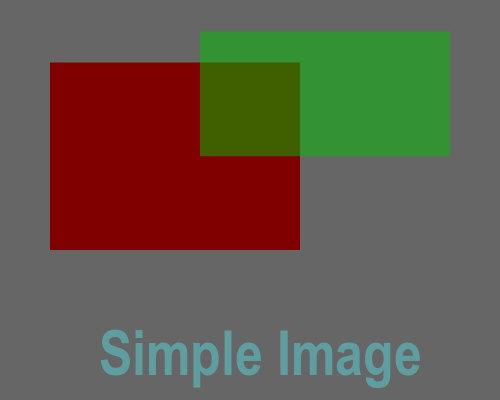# Getting started with generativepy

Martin McBride, 2020-07-26
Categories generativepy generative art

generativepy is a Python library. You can create images or videos by writing a Python script that calls the library. In this section we will look at a very simple example.

## Source code

The code for thos example can be found on github, in the examples/start folder. The file is called simpleimage.py:

from generativepy.drawing import makeImage, setup, text
from generativepy.color import Color

def draw(ctx, pixel_width, pixel_height, frame_no, frame_count):

setup(ctx, pixel_width, pixel_height, width=5, background=Color(0.4))

ctx.set_source_rgba(*Color(0.5, 0, 0))
ctx.rectangle(0.5, 0.5, 2.5, 1.5)
ctx.fill()

ctx.set_source_rgba(*Color(0, 0.75, 0, 0.5))
ctx.rectangle(2, 0.25, 2.5, 1)
ctx.fill()

text(ctx, "Simple Image", 1, 3, size=0.5, color=Color('cadetblue'), font='Arial')

makeImage("/tmp/simpleimage.png", draw, 500, 400)


## Structure

We first import various modules from the generativepy library.

Then we define a draw function. This does the actual drawing of the page.

Finally we call makeImage. This is the function that creates the image, calling our draw function to do the drawing.

## makeImage

Here is the call to makeImage:

makeImage("/tmp/simpleimage.png", draw, 500, 400)


The makeImage function accepts several parameters:

• The full pathname of the PNG file where the image will be stored.
• The draw function that will be used to draw the image.
• The width and height of the final image, in pixels.

Notice that we use draw rather draw(). This passes a function object into makeImage. It can be called later to do the drawing.

Here is what makeImage does:

• Creates a Pycairo surface of the required size.
• Creates a Pycairo context for the surface.
• Calls the draw function it has been given, to draw on the surface.
• Saves the completed image as a PNG file.

In Pycairo, a surface is a thing you draw on - you can think of it as being like a piece of paper. The context is the thing that does the drawing - you could think of it as being like an automated artist. You tell it what to draw on the paper, and it draws it.

In generativepy, you don't need to worry about the surface, you just need to write a draw function that tells the context what to draw.

## draw

The draw function is where you create your image. Here it is:

def draw(ctx, pixel_width, pixel_height, frame_no, frame_count):

setup(ctx, pixel_width, pixel_height, width=5, background=Color(0.4))

ctx.set_source_rgba(*Color(0.5, 0, 0))
ctx.rectangle(0.5, 0.5, 2.5, 1.5)
ctx.fill()

ctx.set_source_rgba(*Color(0, 0.75, 0, 0.5))
ctx.rectangle(2, 0.25, 2.5, 1)
ctx.fill()

text(ctx, "Simple Image", 1, 3, size=0.5, color=Color('cadetblue'), font='Arial')


The function takes 5 parameters:

• ctx is the Pycairo context that we will draw on.
• pixel_width is the width of the image (it is the same value that we passed in to the makeImage function before).
• pixel_height is the height of the image.
• frame_no is used when we create image sequences (for videos or animated GIFs) - it will always be zero when we use makeImage.
• frame_count is the number of images in the sequence - it will always be one when we use makeImage.

Within the draw function we can draw whatever we want, using the full power of Pycairo (and it is pretty powerful). generativepy also provides a few utility functions to do common tasks. These are entirely optional, they don't do anything that can't be done with raw Pycairo, but you might find them convenient sometimes.

Here is the image the code creates:The first thing we call is setup. This is a generativepy function that sets up the page. It is optional but quite useful:

    setup(ctx, pixel_width, pixel_height, width=5, background=Color(0.4))


The first three parameters are just the values that were passed in to draw.

The width value scales our drawing system. Although the output page is 500 pixel wide, we have told Pycairo that we want to specify all our coordinates in a user space where the page is 5 units wide. So each unit corresponds to 100 pixels. the same scaling is applied to the height, so the 500x400 image has a user space size of 5x4 units.

The background parameter sets the image background colour. We have used Color(0.4) (using the generativepy Color object), which gives a 40% grey.

The next code draws a rectangle, using pure Pycairo functions:

    ctx.set_source_rgba(*Color(0.5, 0, 0))
ctx.rectangle(0.5, 0.5, 2.5, 1.5)
ctx.fill()


Color with 3 parameters creates an RGB value, in this case 50% red. *Color unpacks the colour into RGBA valuse (A is the alpha value, in other words the transparency, which defaults to 1). This sets the current colour using set_source_rgba. We create a rectangle at location (0.5, 0.5), with size 2.5 by 1.5, all measured in user units. Finally we fill the rectangle with the selected colour.

We do the same again, to create another rectangle:

    ctx.set_source_rgba(*Color(0, 0.75, 0, 0.5))
ctx.rectangle(2, 0.25, 2.5, 1)
ctx.fill()


In this case we have used Color(0, 0.75, 0, 0.5). 4 arguments creates an RGBA colour, with the transparency set to 0.5. This means that you can see the red rectangle through the green rectangle where they overlap.

Finally, we draw some text:

    text(ctx, "Simple Image", 1, 3, size=0.5, color=Color('cadetblue'), font='Arial')


The text function is another generativepy function. It draws the text at point (1, 3), using an Arial font size 0.5 in user units.

Notice tha the colour this time is set to cadetblue - this is a CSS named colour.